Courses

# IIT JAM Chemistry Mock Test 1

## 60 Questions MCQ Test MOCK Test Series for IIT JAM Chemistry | IIT JAM Chemistry Mock Test 1

Description
This mock test of IIT JAM Chemistry Mock Test 1 for Chemistry helps you for every Chemistry entrance exam. This contains 60 Multiple Choice Questions for Chemistry IIT JAM Chemistry Mock Test 1 (mcq) to study with solutions a complete question bank. The solved questions answers in this IIT JAM Chemistry Mock Test 1 quiz give you a good mix of easy questions and tough questions. Chemistry students definitely take this IIT JAM Chemistry Mock Test 1 exercise for a better result in the exam. You can find other IIT JAM Chemistry Mock Test 1 extra questions, long questions & short questions for Chemistry on EduRev as well by searching above.
QUESTION: 1

Solution:
QUESTION: 2

Solution:
QUESTION: 3

### Aluminium-25 decay by emitting a positron. The species immediately produced has:

Solution:
QUESTION: 4

The temperature coefficient of two reactions are 2 and 3 respectively. Which would be correct for these reactions?

Solution: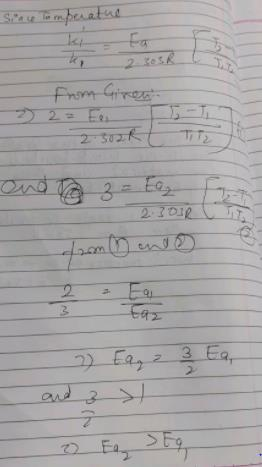QUESTION: 5

Which of the following amino acid is hydrophobic?

Solution:
QUESTION: 6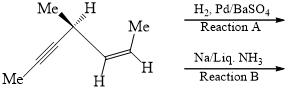Solution:
QUESTION: 7

Arrange the following in decreasing order of stretching frequency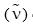of C-O bond (cm–1):
(I) Mo(CO)3(NMe3)3              (II) Mo(CO)3[P(OPh)3]3
(III) Mo(CO)3(PMe3)3            (IV) Mo(CO)3(PCl3)3

Solution:
QUESTION: 8

Which of the following form stable for CN—CH2—CH2—CN?

Solution:
QUESTION: 9

If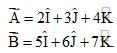Given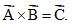Which of the following holds true?

Solution:
QUESTION: 10

By Cannizaro reaction A changes to B and C as given. Hence, A is: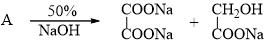Solution:
QUESTION: 11

Emf of Cd-cell is 1.018 V at 25°C. The temperature coefficient of cell is –5.2 × 10–5 VK–1. How cell temperature will change during operation?

Solution:
QUESTION: 12

Match the correctly:
Carbonyl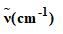(W) 12C O16                                           (k) 2160

(X) 13C O16                                            (l) 1830

(Y) 13C O17                                            (m) 1951

(Z) 12C O17                                              (n) 2106

Solution:
QUESTION: 13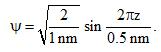Calculate En if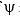represents state of a particle in 1-D box of length 1nm:

Solution:
QUESTION: 14

The given carbocation rearrangement into: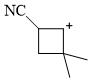Solution:
QUESTION: 15

The self-ionization constant for pure formic, acid,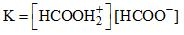has been estimated as 10–6 at room temperature. The density of formic acid is 1.15 g/cm3. The percentage of formic acid molecules in pure formic acid are converted to formate ion:

Solution:
QUESTION: 16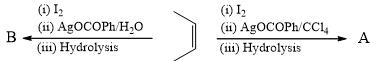Products A and B are:

Solution:
QUESTION: 17

25 mL of hydrogen and 18 mL of Iodine when heated in closed container, produced 30.8 mL of HI at equilibrium. Calculate degree of dissociation of HI at same temperature:

Solution:
QUESTION: 18

For a surface inactive substance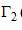(excessive concentration of solute per unit area of surface) is essentially:

Solution:
QUESTION: 19

Which of the following does not show facial & meridonial isomerism?

Solution:
QUESTION: 20

The plane(s) which show allowed reflection in both bcc and fcc lattices is/are:
(I) 100                               (II) 111                                 (III) 110

Solution:
QUESTION: 21

Consider μ1, μ2, μ3 are dipole moments of Pyrrole, Furan, Thiopene respectively. Which of the following holds true:

Solution:
QUESTION: 22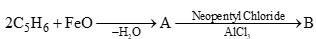The product B is:

Solution:
QUESTION: 23

Which of the following conversion take place in bourdon tubes?

Solution:

In bourdon tubes converts input pressure into displacement and displacement of the needle will be directly proportional to input pressure.

QUESTION: 24

Which of the following is true about Osazone formation in carbohydrates?

Solution:
QUESTION: 25

If bond length of CO bond in carbon monoxide is 1.128 Å, then, what is the value of CO bond length in Fe(CO)5?

Solution:
QUESTION: 26

Consider borax. Chemical formula of which is Na2B4O7. 10H2O. Choose the incorrect statement:

Solution:
QUESTION: 27

Which of the following will give different product on hydrolysis (among others)?

Solution:
QUESTION: 28

1H NMR spectrum of a compound with molecular formula C4H9NO2 shows:
d 5.30 (broad, 1H); d 4.10 (q, 2H); d 2.80 (d, 3H); d 1.20 (t, 3H). The structure of compound that is consistence with the above data is:

Solution:
QUESTION: 29

How are the following compounds related?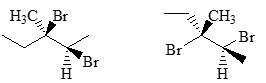Solution:
QUESTION: 30

If sin2q = 0.1198, 0.2395, 0.3588, 0.4793, 0.5984. Find lattice type:

Solution:
*Multiple options can be correct
QUESTION: 31

Which of the following conversions is/are correctly presented?

Solution:
*Multiple options can be correct
QUESTION: 32

Select the correct statement with respect to alkali metals.

Solution:
*Multiple options can be correct
QUESTION: 33

Choose the correct statement/s:

Solution:
*Multiple options can be correct
QUESTION: 34

Incorrect statement regarding above compounds: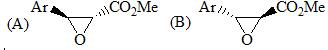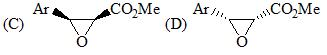(I) A and C are enantiomer           (II) B and D are diastereomers
(III) C and D are enantiomers       (IV) A and B are homomer

Solution:
*Multiple options can be correct
QUESTION: 35

Choose the correct statement/s among following:

Solution:
*Multiple options can be correct
QUESTION: 36

Select the correct statements.

Solution:
*Multiple options can be correct
QUESTION: 37

Which statement is incorrect about the following reaction?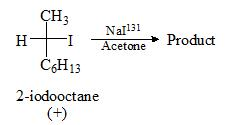Solution:
*Multiple options can be correct
QUESTION: 38

Which of the following is/are correct in reference to RNA?

Solution:
*Multiple options can be correct
QUESTION: 39

Which of following conversions is/are correct in reference to peri-cyclic reactions?

Solution:
*Multiple options can be correct
QUESTION: 40

Which of the following conversion is/are incorrectly presented?

Solution:
*Answer can only contain numeric values
QUESTION: 41

What is the spin multiplicity of Fischer carbene?

Solution:
*Answer can only contain numeric values
QUESTION: 42

How many signals are obtained for the following compound in 1H NMR spectroscopy?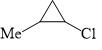Solution:
*Answer can only contain numeric values
QUESTION: 43

M/O2 (where M = Metal center) binding ratio in Hemocyanin is:

Solution:
*Answer can only contain numeric values
QUESTION: 44

Given is the ester hydrolysis reaction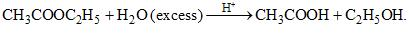Order of reaction is __________?

Solution:
*Answer can only contain numeric values
QUESTION: 45

The number of S–S bonds, in sulphur trioxide trimer (S3O9) is :

Solution:
*Answer can only contain numeric values
QUESTION: 46

Number of 2c -2e- bonds in Al2(CH3)6 :

Solution:
*Answer can only contain numeric values
QUESTION: 47

Root mean square velocity of H2 molecule having density 0.09 gL–1 at 1 atm is x × 103 ms-1 then    what is the value of x? (rounded up to first decimal place)

Solution:
*Answer can only contain numeric values
QUESTION: 48

100 ml of unknown reducing agent is reacted with 500 mL, 0.1 M excess I2 solution. If remaining I2 required 0.1 M, 200 mL Na2S2O3 solution for complete neutralization. Calculate normality of unknown reducing agent.

Solution:
*Answer can only contain numeric values
QUESTION: 49

Consider the silicate Ba2NaxSi5O15. What is the value of ‘x’?

Solution:
*Answer can only contain numeric values
QUESTION: 50

A sample of rock from moon contains equal number of atoms of uranium and lead. The age of the rock would be (in years, rounded up to first decimal place, in the multiple of 109) of the mineral. (Half-life of 238U is 4.5 × 109 years):

Solution:
*Answer can only contain numeric values
QUESTION: 51

If one of the bonds in NH3 molecule has 22% s-character, how much percentage of it is expected to be present in lone pair? (rounded up to first decimal place)

Solution:
*Answer can only contain numeric values
QUESTION: 52

Depression of freezing point of 0.01 molal aq. CH3COOH solution is 0.02046°. 1 molal urea    solution freezes at – 1.86°C. Assuming molality equal to molarity, pH (rounded up to first decimal place) of CH3COOH solution is?

Solution:
*Answer can only contain numeric values
QUESTION: 53

A solution made up to be 0.0100 M Co(NO3)2 and 0.0200 M N2H4 was found to have an equilibrium concentration of [Co2+] is 6 × 10–3 M. Assuming that the only complex formed was Co(N2H4)2+, what is the apparent Kf for complex formation? (rounded up to two decimal place)

Solution:
*Answer can only contain numeric values
QUESTION: 54

Number of metal-metal bond in [Re2Cl4(PPhMe2)4] is?

Solution:
*Answer can only contain numeric values
QUESTION: 55

The pKa values for the three ionizable groups, X, Y and Z of glutamic acid are 4.3, 9.7 and 2.2 respectively: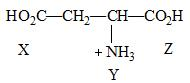The isoelectric point for the amino acid is:

Solution:
*Answer can only contain numeric values
QUESTION: 56

Find the value of lmax in (nm) in following compound.
Given: Base value of Heteroannular component = 214 nm
Homoannular component = 253 nm
Increment for each extra double bond = 30 nm
Exocyclic bond = 5 nm; Alkyl/Ring residues = 5 nm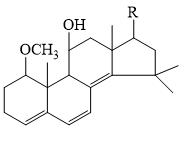Solution:
*Answer can only contain numeric values
QUESTION: 57

How many of the following ores contain both iron & copper?
Cuprite, Chalcocite, Chalcopyrite, Malachite, Copper Glance.

Solution:
*Answer can only contain numeric values
QUESTION: 58

What is the group number of the element having electronic configuration [Xe]4F145S2?

Solution:
*Answer can only contain numeric values
QUESTION: 59

Among the following, the number of molecules that possess C2 axis of symmetry is ________: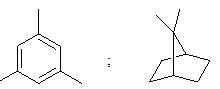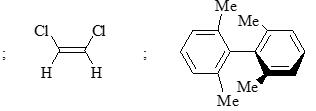BF3 ; BGCI3 ; 2,5 -dimethythiophene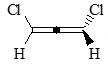Solution:
*Answer can only contain numeric values
QUESTION: 60

Calculate the specific rotations (in 0°C) of the following sample taken at 25°C using the sodium D line. 1.00 g of sample is dissolved in 20.0 mL of ethanol. Then 5.00 mL of this solution is placed in a 20.0 cm polarimeter tube. The observed is 1.25° counterclockwise.

Solution: